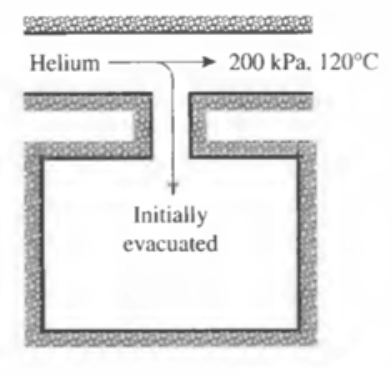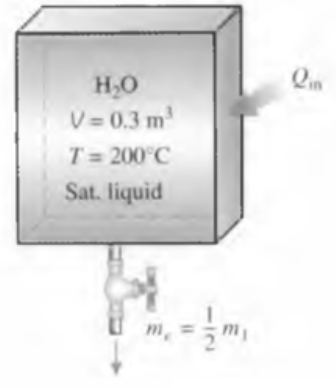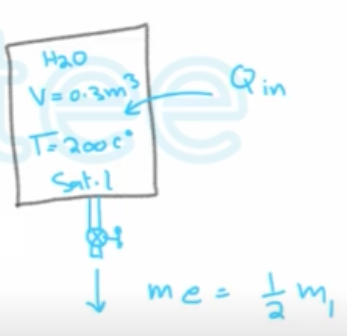Need Help?

Subscribe to Thermodynamics

###### \${selected_topic_name}
• Notes

$\begin{array}{l}{\text { A rigid, insulated tank that is initially evacuated is connected through a valve to a supply line }} \\ {\text { that carries helium at } 200 \mathrm{kPa} \text { and } 120^{\circ} \mathrm{C} \text { . Now the valve is opened, and helium is allowed }} \\ {\text { to flow into the tank until the pressure reaches } 200 \mathrm{kPa} \text { , at which point the value is closed. }}\end{array}$

$\begin{array}{l}{\text { Determine the flow work of the helium in the supply line and the final temperature }} \\ {\text { of the helium in the tank. }}\end{array}$$A-2(a) \rightarrow R=2.0769,$$C v=3.1156, \quad C \rho$

(in) $P_{i n}=200 \mathrm{KPa}\quad ,\quad T_{i n}=120 c^{\circ} = 393k$

$\text { PV } \longrightarrow \text { Pv }$

$\text { I.G } \rightarrow \quad p v=R T=20769*393=816.2 kJ/kg$

$\text { (flow work) }$

$h_{i n}=C p \ T_{i n}=5.1926*393=2040.69 kJ/kg$

(2) $P_{2}=200 \mathrm{KPa}$

$m_{i n}-m_{o u t}=m_2-m_1\rightarrow m_{in}=m_2$

$Q_{i n}-Q_{out}+\left(w_{i n}-w_{out}\right)+(m_{i n} \theta_{i n}-m_{out})=m_2 v_2-m_1 v_1$

$\rightarrow m_{in} h_{in}=m_2 u_2\rightarrow h_{in}=u_2=2040.69kJ/kg$

$U_{2}=C_{V} T_{2} \rightarrow T_{2}=U_{2}/C_V$

$\rightarrow T_2=\frac{2040 .69}{3.1156}=655 \mathrm{k}$

$\text { A } 0.3-m 3 \text { rigid tank is filled with saturated liquid water at } 200^{\circ} \mathrm{C} \text { . A valve at the bottom of the tank is }$

$\begin{array}{l}{\text { opened, and liquid is withdrawn from the tank. Heat is transferred to the water such that }} \\ {\text { the temperature in the tank remains constant. Determine the amount of heat that must }} \\ {\text { be transferred by the time one-half of the total mass has been withdrawn. }}\end{array}$$A-4,5,6$

$\left.\begin{array}{l}{T_{1}=200^{\circ} C} \\ {Sat . L}\end{array}\right]\rightarrow$$V_{1}=V_{f} @ 200 c^{ \circ}=0.001157$

$U_{1}=V_{f} @ {200} c^{\circ}=850.46$

$\left.\begin{array}{l}{T_{2}=200^{\circ} C} \\ {Sat. L}\end{array}\right]\rightarrow h_e=h_{f@200 c^{\circ}}=852.26$

$m_{i n}- m_{out}=\Delta m_{s y s}$

$m_{in}- m_{out}=m_{1}-m_{2}$

$m_ e=m_{1}-m_{2}$

$E_{in}-E_{out}=\Delta E_{sys}$

$Q_{i n}=m_{e} h_{e}+m_{2} v_{2}-m_{1} v_{1}$

$m_{1}=\frac{V_{1}}{V_{1}}=\frac{0.3}{0.001157}=$$259.4 \mathrm{kg} \rightarrow \mathrm{m_e}=\frac{\mathrm{1}}{2} \mathrm{m_1}=129.7 \mathrm{kg}$

$V_{2}=\frac{V}{m_{2}}=\frac{6 \cdot 3}{129.7}=0.002313 \mathrm{m}^{3} / \mathrm{kg}$

$X_{2}=\frac{V_{2}-V_{f}}{V_{fg}}=\frac{0.002313-0.001157}{0.12721-0.001157}=0.009171$

$\left.\begin{array}{l}{T_{2}=200 c^{\circ}} \\ {X_{2}=0.009171}\end{array}\right]\rightarrow$$V_{2}=V_{f}+X_{2} U_{f g}=850.46+0.009171(1743.7)$

$∴ V_{2}=866.5 kJ/kg$

$\rightarrow Q=(129.7)(852.26)+(129.7)(866.46)-(259.4)(850.46)=2308kJ$